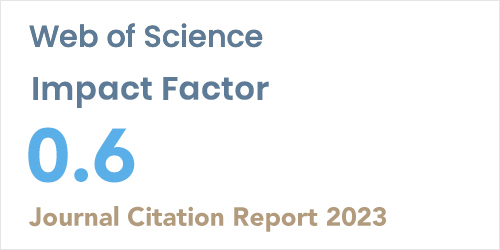ISSN: 1304-7191 | E-ISSN: 1304-7205
Volume: 41 Issue: 4 Year: 2023Design of experiments optimization application in physics: a case study of the damped driven pendulum experiment
1Gaziantep Islam, Science and Technology University, Industrial Engineering Department, Gaziantep, Turkey
2Bursa Technical University, Industrial Engineering Department, Bursa, Turkey
Sigma J Eng Nat Sci 2021; 39(3): 322-330 DOI: 10.14744/sigma.2021.00020

#### Abstract

The design of experiment (DoE) approach developed for experiments requiring cost and time is applied in many disciplines. Unfortunately, the insufficient use of the DoE technique in physics led to the emergence of this study. This study aims to demonstrate the applicability of the DoE technique in the field of physics with a case study. The most widely used full factorial experimental design was used for the damped driven pendulum case study. Length (m), dumpling (Newton), and mass (kg) as independent and energy (joule) as dependent variables were defined in this study to apply the DoE approach. As a result of the statistical analyses in DoE, optimization models were created, and optimum values were obtained for the case study. The experiment performed was proved to be statistically significant and valid by calculating the R-square as 0.97. The value of the objective function is calculated as 4.058 (joule). The optimum values for length, dumping, and mass was calculated as 2.719 m, 2.485(Newton), and 2.895 kg, respectively. In conclusion, this study will contribute to the literature to guide the researchers who spend a lot of time in experimental labs and have problems with experiment costs.